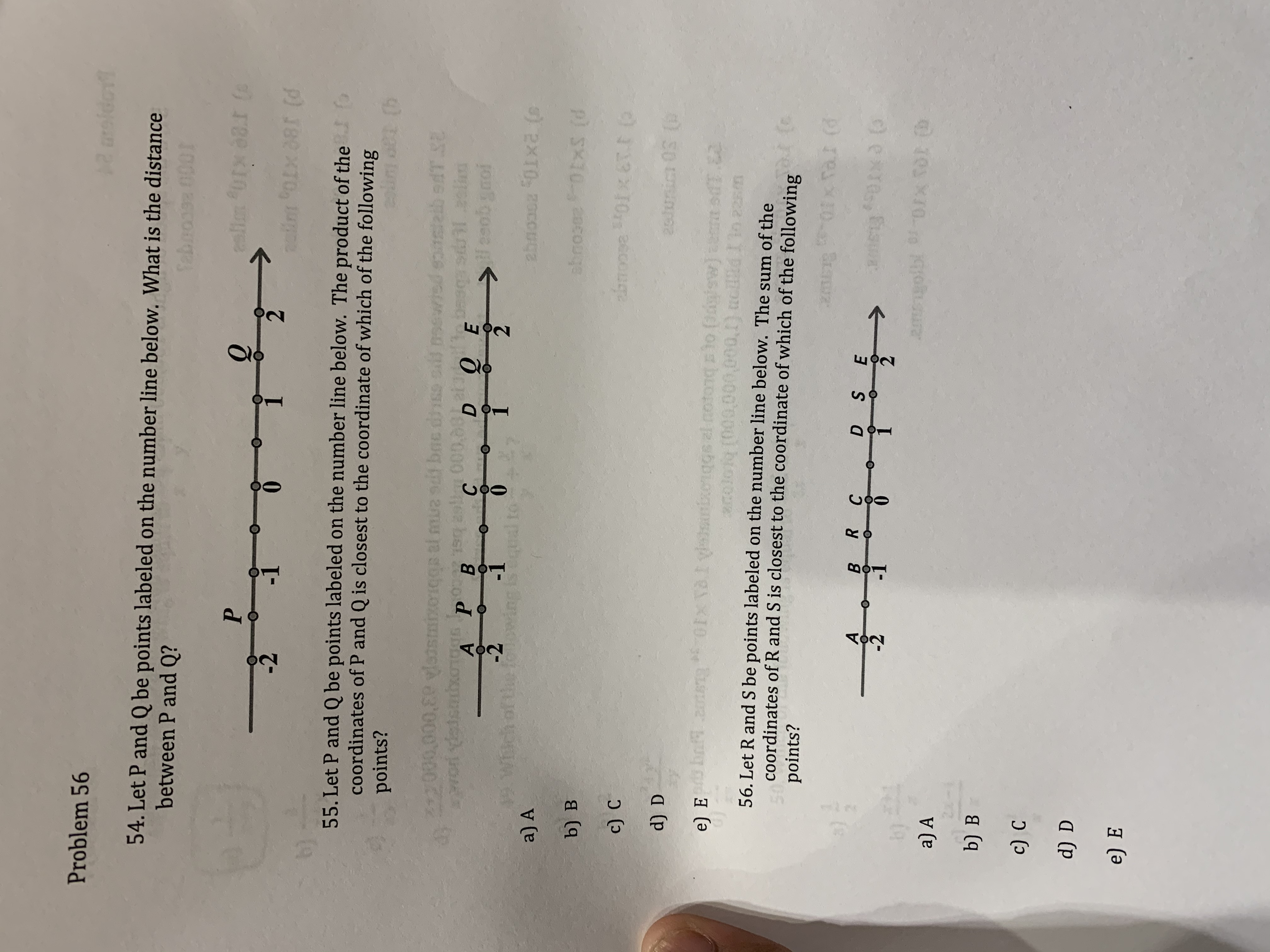# Problem 5612aroido54. Let P and Q be points labeled on the number line below. What is the distancebetween P and Q?Sebnoose 0001lln 401x 88.1 (oPnuin oLx a8r (d08T (dfo55. Let P and Q be points labeled on the number line below. The product of thecoordinates of P and Q is closest to the coordinate of which of the followingpoints?OAnlibsdtE9031 alinHcoob gnoited sins213000 000,89 l mua suf bac dsBHVO dedso er as000.08t tobege, 130PВО ЕDEСА-1-2the021ingqual toahnoc 01x (sa) Asbooze 0xS (db) вbroose 0x ET.c) C2J0nic 03 (d) Dba n IX v xenggs a notong to (dl tsdT.E2n artsi(Ddy'w]hasne*nolofo [00000,000 1) cBBdIT56. Let R and S be points labeled on the number line below. The sum of thecoordinates of R and S is closest to the coordinate of which of the followinge) Epoints?01x Tal (12meDEF0Ixd2-12exx., Eis2 b0FX TOl D193 K10.22ITGTEa) Ab) Bc) Cd) De) ЕSoROBO

Question
33 views

Need help on 54 and 55help_outlineImage TranscriptioncloseProblem 56 12 aroido 54. Let P and Q be points labeled on the number line below. What is the distance between P and Q? Sebnoose 0001 lln 401x 88.1 (o P nuin oLx a8r (d 08T (d fo 55. Let P and Q be points labeled on the number line below. The product of the coordinates of P and Q is closest to the coordinate of which of the following points? OAnlibsdtE 9031 alin Hcoob gnoi ted sins 213000 000,89 l mua suf bac ds B HVO dedso er as000.08t tobe ge, 130 P В О Е D E С А -1 -2 the 0 2 1 ing qual to ahnoc 01x (s a) A sbooze 0xS (d b) в broose 0x ET. c) C 2J0nic 03 ( d) D ba n IX v xenggs a notong to (dl tsdT.E2 n artsi (Ddy'w]hasne *nolofo [00000,000 1) cBBdIT 56. Let R and S be points labeled on the number line below. The sum of the coordinates of R and S is closest to the coordinate of which of the following e) E points? 01x Tal (1 2me D E F0Ixd 2 -1 2 exx., Eis 2 b0FX TOl D 193 K10. 22ITGTE a) A b) B c) C d) D e) Е So RO BO fullscreen
check_circle

Step 1

54. The coordinates of point P is -1.5  and Q is 1.5

Then distance between P and Q is 1.5-(-1.5)=3

Step 2

55. The product of the coordinates of point P and Q is -1.5*1.5 is -2.25

Thu...

### Want to see the full answer?

See Solution

#### Want to see this answer and more?

Solutions are written by subject experts who are available 24/7. Questions are typically answered within 1 hour.*

See Solution
*Response times may vary by subject and question.
Tagged in

### Algebra